# Sprinter

Sprinter runs the relay 4 x 400 m to the handover at a speed of 42 km/h. A second runner is at the start of the handover area 20 m long and runs when it is the first sprinter at a distance of 10 m. Calculate the speed at which the second runner must run to the handover that occurred at the end of the handover area. Consider that the speed of both runners is constant.

v =  28 km/h

### Step-by-step explanation: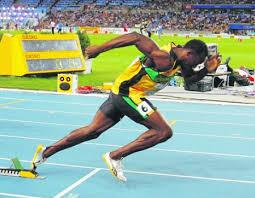Did you find an error or inaccuracy? Feel free to write us. Thank you!Tips to related online calculators
Check out our ratio calculator.
Do you have a linear equation or system of equations and looking for its solution? Or do you have a quadratic equation?
Do you want to convert velocity (speed) units?
Do you want to convert time units like minutes to seconds?

## Related math problems and questions:

• Two runners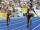Two runners ran simultaneously towards each other from locations distant 34.6 km. The average speed of the first runner was 1/5 higher than the average speed of the second runner. How long should each ran a 34.6 km, if you know that they meet after 67 min
• Runner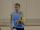Peter ran a speed of 12.4 km / h. After 45 minutes running he had a hiatus. The track is 31 km long. How many kilometers he still to run if he still running at the same speed?
• Runner 2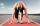Marian runs 12 meters in 8 seconds. How far would Marian run for 70 seconds if he still runs at the same pace?
• Bob and Bobek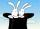At 10h 20min runs Bobek from the hat at average speed 12 km/h. At what time must Bob run out at speed 18 km/h to catch him 9 kilometers from the hat?
• Constant motionThe car has undergone constant motion 120 km in 1 hour 30 minutes. Determine its average speed. Determine how long the car drove in the first 20 minutes and 50 minutes from the start of the move.
• Two citiesCities A and B are 200 km away. At 7 o'clock from city A, the car started at an average speed of 80 km/h, and from B at 45 min later, the motorcycle is started at an average speed of 120 km/h. How long will they meet, and at what distance from point A wil
• Pedestrian and the cyclist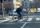Pedestrian and cyclist went against each from the two cities that are distant 40 km. Pedestrian started 30 minutes before the cyclist and walk an average speed of 5 km/h. What speed went cyclist when met after 120 minutes from the start of the pedestrian?
• Motion 2At 10 o'clock started from place A car speed at 90 km/h. Twenty minutes after starting another car from place B at a speed 60 km/h . Distance places A and B is 120 kilometers. What time and where do they meet?
• Cyclist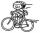The cyclist goes uphill 10 km for 50 minutes and downhill minutes for 29 minutes, both applied to the pedals the same force. How long he pass 10 km by plane?
• Car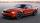The car goes from point A to point B at speed 86 km/h and back 53 km/h. If it goes there and back at speed 67 km/h trip would take 10 minutes shorter. What is the distance between points A and B?
• Double-track line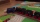A 160 m long passenger train runs on a double-track line in one direction at a constant speed of 54 km/h, and a 240 m long express train in the opposite direction. a) How fast is the express train if passing the passenger train driver for 6 s? b) How long
• The escalator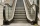I run up the escalator at a constant speed in the stairs' direction and write down the number of steps A we climbed. Then we turn around and run it at the same constant speed in the opposite direction and write down the number of steps B that I climbed. I
• Cars motion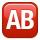From point A car start at the speed of 90 km/h. Against it departs from the point B at the same time another car at 60 km/h. For how long and where they met if the distance between A and B is 180 km?
• Racer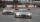The first racer run at a speed 20 m/s and is 750 meters before the finish and ahead 79 meters before second competitor. At what speed must run the second racer to catch first racer at the finish line?
• Car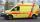The car drove some distance in 3 hours and 20 minutes. If it increases speed by 10 km/h, it goes this distance in 2.5 hours. Calculate the distance.
• Peleton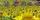The cycling race was run at an average speed of 45 km/h. One cyclist lost with defect 8 minutes. How long and how far he must go at speed 51 km/h to catch the peloton again?
• The ship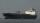The ship went upstream speed of 20 km/h in relation to water. River flows at speed 10 km/h. After half an hour, he stopped and returned downstream to the starting point. How long it took way back when even if downstream speed of ship is 20 km/h in relatio